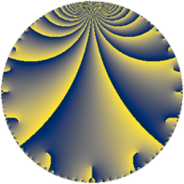Properties

 Label 1089.3.c.bLevel $1089$ Weight $3$ Character orbit 1089.c Analytic conductor $29.673$ Analytic rank $0$ Dimension $4$ CM no Inner twists $4$

Related objects

Newspace parameters

 Level: $$N$$ $$=$$ $$1089 = 3^{2} \cdot 11^{2}$$ Weight: $$k$$ $$=$$ $$3$$ Character orbit: $$[\chi]$$ $$=$$ 1089.c (of order $$2$$, degree $$1$$, minimal)

Newform invariants

 Self dual: no Analytic conductor: $$29.6731007888$$ Analytic rank: $$0$$ Dimension: $$4$$ Coefficient field: $$\Q(\sqrt{-2}, \sqrt{-11})$$ Defining polynomial: $$x^{4} - 2 x^{3} + 11 x^{2} - 10 x + 3$$ Coefficient ring: $$\Z[a_1, \ldots, a_{7}]$$ Coefficient ring index: $$2^{2}$$ Twist minimal: yes Sato-Tate group: $\mathrm{SU}(2)[C_{2}]$

$q$-expansion

Coefficients of the $$q$$-expansion are expressed in terms of a basis $$1,\beta_1,\beta_2,\beta_3$$ for the coefficient ring described below. We also show the integral $$q$$-expansion of the trace form.

 $$f(q)$$ $$=$$ $$q -\beta_{3} q^{2} -7 q^{4} -2 \beta_{1} q^{5} + 7 \beta_{2} q^{7} + 3 \beta_{3} q^{8} +O(q^{10})$$ $$q -\beta_{3} q^{2} -7 q^{4} -2 \beta_{1} q^{5} + 7 \beta_{2} q^{7} + 3 \beta_{3} q^{8} + 22 \beta_{2} q^{10} + 9 \beta_{2} q^{13} + 7 \beta_{1} q^{14} + 5 q^{16} -3 \beta_{2} q^{19} + 14 \beta_{1} q^{20} -6 \beta_{1} q^{23} + 63 q^{25} + 9 \beta_{1} q^{26} -49 \beta_{2} q^{28} -4 \beta_{3} q^{29} -44 q^{31} + 7 \beta_{3} q^{32} -28 \beta_{3} q^{35} + 44 q^{37} -3 \beta_{1} q^{38} -66 \beta_{2} q^{40} -12 \beta_{3} q^{41} -21 \beta_{2} q^{43} + 66 \beta_{2} q^{46} -2 \beta_{1} q^{47} -49 q^{49} -63 \beta_{3} q^{50} -63 \beta_{2} q^{52} + 14 \beta_{1} q^{53} -21 \beta_{1} q^{56} -44 q^{58} -4 \beta_{1} q^{59} + 49 \beta_{2} q^{61} + 44 \beta_{3} q^{62} + 97 q^{64} -36 \beta_{3} q^{65} -88 q^{67} -308 q^{70} + 10 \beta_{1} q^{71} -29 \beta_{2} q^{73} -44 \beta_{3} q^{74} + 21 \beta_{2} q^{76} -9 \beta_{2} q^{79} -10 \beta_{1} q^{80} -132 q^{82} + 28 \beta_{3} q^{83} -21 \beta_{1} q^{86} -24 \beta_{1} q^{89} -126 q^{91} + 42 \beta_{1} q^{92} + 22 \beta_{2} q^{94} + 12 \beta_{3} q^{95} + 70 q^{97} + 49 \beta_{3} q^{98} +O(q^{100})$$ $$\operatorname{Tr}(f)(q)$$ $$=$$ $$4 q - 28 q^{4} + O(q^{10})$$ $$4 q - 28 q^{4} + 20 q^{16} + 252 q^{25} - 176 q^{31} + 176 q^{37} - 196 q^{49} - 176 q^{58} + 388 q^{64} - 352 q^{67} - 1232 q^{70} - 528 q^{82} - 504 q^{91} + 280 q^{97} + O(q^{100})$$

Basis of coefficient ring in terms of a root $$\nu$$ of $$x^{4} - 2 x^{3} + 11 x^{2} - 10 x + 3$$:

 $$\beta_{0}$$ $$=$$ $$1$$ $$\beta_{1}$$ $$=$$ $$\nu^{2} - \nu + 5$$ $$\beta_{2}$$ $$=$$ $$($$$$2 \nu^{3} - 3 \nu^{2} + 19 \nu - 9$$$$)/3$$ $$\beta_{3}$$ $$=$$ $$($$$$4 \nu^{3} - 6 \nu^{2} + 44 \nu - 21$$$$)/3$$
 $$1$$ $$=$$ $$\beta_0$$ $$\nu$$ $$=$$ $$($$$$\beta_{3} - 2 \beta_{2} + 1$$$$)/2$$ $$\nu^{2}$$ $$=$$ $$($$$$\beta_{3} - 2 \beta_{2} + 2 \beta_{1} - 9$$$$)/2$$ $$\nu^{3}$$ $$=$$ $$($$$$-8 \beta_{3} + 19 \beta_{2} + 3 \beta_{1} - 14$$$$)/2$$

Character values

We give the values of $$\chi$$ on generators for $$\left(\mathbb{Z}/1089\mathbb{Z}\right)^\times$$.

 $$n$$ $$244$$ $$848$$ $$\chi(n)$$ $$-1$$ $$1$$

Embeddings

For each embedding $$\iota_m$$ of the coefficient field, the values $$\iota_m(a_n)$$ are shown below.

For more information on an embedded modular form you can click on its label.

Label $$\iota_m(\nu)$$ $$a_{2}$$ $$a_{3}$$ $$a_{4}$$ $$a_{5}$$ $$a_{6}$$ $$a_{7}$$ $$a_{8}$$ $$a_{9}$$ $$a_{10}$$
604.1
 0.5 + 0.244099i 0.5 + 3.07253i 0.5 − 0.244099i 0.5 − 3.07253i
3.31662i 0 −7.00000 −9.38083 0 9.89949i 9.94987i 0 31.1127i
604.2 3.31662i 0 −7.00000 9.38083 0 9.89949i 9.94987i 0 31.1127i
604.3 3.31662i 0 −7.00000 −9.38083 0 9.89949i 9.94987i 0 31.1127i
604.4 3.31662i 0 −7.00000 9.38083 0 9.89949i 9.94987i 0 31.1127i
 $$n$$: e.g. 2-40 or 990-1000 Significant digits: Format: Complex embeddings Normalized embeddings Satake parameters Satake angles

Inner twists

Char Parity Ord Mult Type
1.a even 1 1 trivial
3.b odd 2 1 inner
11.b odd 2 1 inner
33.d even 2 1 inner

Twists

By twisting character orbit
Char Parity Ord Mult Type Twist Min Dim
1.a even 1 1 trivial 1089.3.c.b 4
3.b odd 2 1 inner 1089.3.c.b 4
11.b odd 2 1 inner 1089.3.c.b 4
33.d even 2 1 inner 1089.3.c.b 4

By twisted newform orbit
Twist Min Dim Char Parity Ord Mult Type
1089.3.c.b 4 1.a even 1 1 trivial
1089.3.c.b 4 3.b odd 2 1 inner
1089.3.c.b 4 11.b odd 2 1 inner
1089.3.c.b 4 33.d even 2 1 inner

Hecke kernels

This newform subspace can be constructed as the kernel of the linear operator $$T_{2}^{2} + 11$$ acting on $$S_{3}^{\mathrm{new}}(1089, [\chi])$$.

Hecke characteristic polynomials

$p$ $F_p(T)$
$2$ $$( 11 + T^{2} )^{2}$$
$3$ $$T^{4}$$
$5$ $$( -88 + T^{2} )^{2}$$
$7$ $$( 98 + T^{2} )^{2}$$
$11$ $$T^{4}$$
$13$ $$( 162 + T^{2} )^{2}$$
$17$ $$T^{4}$$
$19$ $$( 18 + T^{2} )^{2}$$
$23$ $$( -792 + T^{2} )^{2}$$
$29$ $$( 176 + T^{2} )^{2}$$
$31$ $$( 44 + T )^{4}$$
$37$ $$( -44 + T )^{4}$$
$41$ $$( 1584 + T^{2} )^{2}$$
$43$ $$( 882 + T^{2} )^{2}$$
$47$ $$( -88 + T^{2} )^{2}$$
$53$ $$( -4312 + T^{2} )^{2}$$
$59$ $$( -352 + T^{2} )^{2}$$
$61$ $$( 4802 + T^{2} )^{2}$$
$67$ $$( 88 + T )^{4}$$
$71$ $$( -2200 + T^{2} )^{2}$$
$73$ $$( 1682 + T^{2} )^{2}$$
$79$ $$( 162 + T^{2} )^{2}$$
$83$ $$( 8624 + T^{2} )^{2}$$
$89$ $$( -12672 + T^{2} )^{2}$$
$97$ $$( -70 + T )^{4}$$